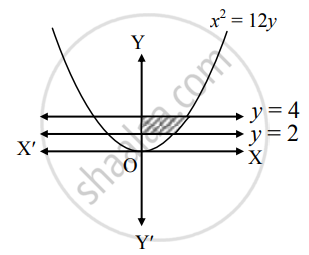# Find the area of the region bounded by the curve x2 = 12y, the Y−axis and the given lines y = 2, y = 4, x ≥ 0 - Mathematics and Statistics

Sum

Find the area of the region bounded by the curve x2 = 12y, the Y−axis and the given lines y = 2, y = 4, x ≥ 0

#### Solution

Let A be the required area.

Consider the equation x2 = 12y∴ A = int_2^4 x  "d"y

= int_2^4 sqrt(12y)  "d"y

= 2sqrt(3) [(y^(3/2))/(3/2)]_2^4

= (4sqrt(3))/3 [(4)^(3/2) - (2)^(3/2)]

= 4/sqrt(3) (8 - 2sqrt(2))

= 8/sqrt(3) (4 - sqrt(2)) sq.units

Concept: Area Bounded by the Curve, Axis and Line
Is there an error in this question or solution?